# const - JS | lectureData Structures, Modern Operators & Strings

Rest Pattern and Parameters

Moving on, let's now talk about the rest pattern and also rest parameters. The rest pattern looks exactly like the spread operator. It has the same syntax with the three dots, but it actually does the opposite of the spread operator.

That doesn't seem to make much sense, but let me explain. So remember that we used the spread operator to build new arrays or pass multiple values into a function. Those are the two use cases of the spread operator, and in both cases, we use the spread operator to expand an array into individual elements.

Now, the rest pattern uses the same syntax, however, to collect multiple elements and condense them into an array. That's the opposite of spread. The spread operator is to unpack an array while the rest operator packs elements into an array. And since that sounds confusing, let's write some code, and let's start by exploring the use case of building arrays.

script.js
``````/**
* We wanted to create a new array based on an existing array
* reason why we used the spread operator to expand the array [3, 4]
*/
const arr = [1, 2, ...[3, 4]];``````

From the above code snippet, we know that it is the spread syntax because we are using it (the three dots) on the right-hand side of the assignment operator. However, we can also use it on the left-hand side of the assignment operator together with destructuring. So now we're mixing everything here 😂 but bear with me here.

So let's say that we are destructuring the below array.

script.js
``const [] = [1, 2, 3, 4, 5];``

Let's say I want to take the first element and store it in variable `a`

script.js
``const [a] = [1, 2, 3, 4, 5];``

Then, let's take the second element and store it in variable `b`

script.js
``const [a, b] = [1, 2, 3, 4, 5];``

And now we can use the rest pattern.

script.js
``````/**
* Same syntax as the spread operator
*
* It is the rest syntax because it is on the left-hand side
* of the assignment operator.
*/

const [a, b, ...others] = [1, 2, 3, 4, 5];

console.log(a, b, others);``````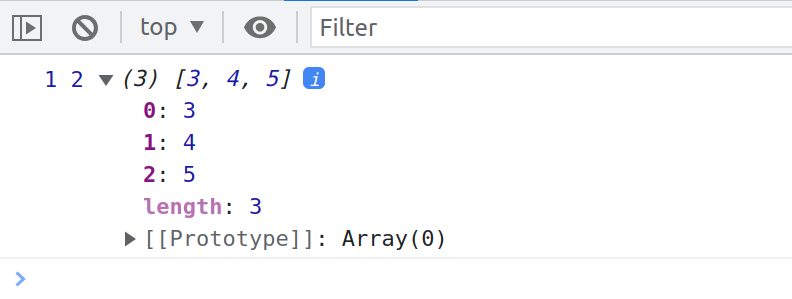You can see that we have one, two, and then basically the rest of the elements that we did not select into the `a` and the `b` variables.

The question now is, what is happening here? Well, just like before, the first and the second elements become `a` and `b`. respectively. But then comes the rest pattern, and so it's called rest because it will take the rest of the elements. That is, the remaining elements of the array and then put them into a new array, and in this case, we call this array `others`.

So as I said in the beginning, the rest pattern collects the unused elements in the destructuring assignment. Let's see another example, and this will show you that we can use the three dots on both sides of the assignment operator.

Let's say that we have this array which will be the entire menu.

script.js
``````/**
* RIGHT OF EQUAL OPERATOR (SPREAD)
* We are expanding bothe the starter and main menu arrays in a whole new array
*
*
* LEFT OF EQUAL OPERATOR (DESTRUCTURING AND REST)
* 👉 [pizza, , risotto, ...otherFood]
* We selected the first and third element and the remaining
* elements with be in the otherFood variable which is an array of
* unselected elements during destructuring
*/

const [pizza, , risotto, ...otherFood] = [
];

console.log(pizza, risotto, otherFood);``````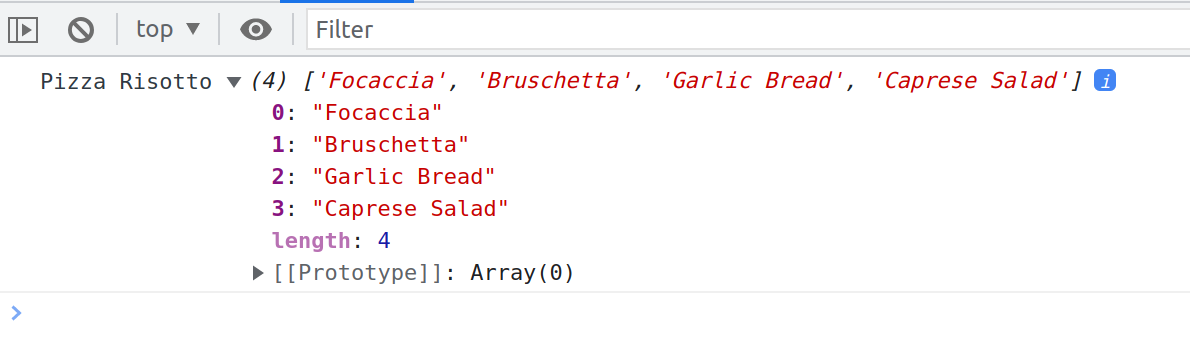As mentioned in the above comments, you can see that we get the string Pizza, the string Risotto, and all the other elements that we didn't select previously into their own variables. Note here that the rest syntax collects all the array elements after the last variable. So, in this case, here is `risotto`. It does not include any skipped elements. It's really just the rest of the elements.

Hence, for that reason, the rest pattern must always be the last in the destructuring assignment because otherwise, how will JavaScript know when it should collect the rest of the array? For example, we could not do this

script.js
``````const [pizza, , risotto, ...otherFood, bread] = [  ...restaurant.mainMenu,
];``````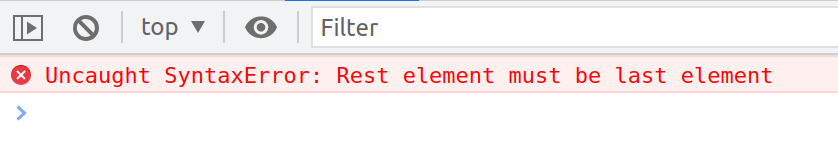You can see that the error message is very explicit. The rest element must be the last element. For the same reason, there can only ever be one rest in any destructuring assignment. And now, let's do the same in objects because it also works indeed in objects.

The difference, then, is that the remaining elements will be collected into a new object and not into a new array. Let's now work here with our opening hours, and let's say that we want to select only Saturday, and then the rest should go into a new object, the `weekdays`.

script.js
``````const { sat, ...weekdays } = restaurant.openingHours;
console.log(weekdays);``````We get exactly what we were expecting. It is an object only containing Friday and Thursday, and again, that's because we already took Saturday into its own variable before, and so this then collected the rest of the properties into its own new object.

Now we know how the rest pattern works to collect elements in a destructuring assignment. Remember that for the spread operator, the second use case was to pass multiple arguments into a function simultaneously.

That's exactly what we did in our real world example in the previous lecture with this ingredients array, remember? We had an array, and then we expanded all of its elements to pass them as individual arguments of the function and so now, as you can guess, the rest operator can do the opposite, and so let's now write an example, function for that.

Let's say we want to write a function called `add`, which can add any arbitrary amount of arguments. That is, we want to be able to do the following:

script.js
``````/**
* We are of course not going to specify all these arguments!!!
*/
add(8, 2, 5, 3, 2, 1, 4);``````

To do that, we will use the rest pattern, and in this case, it is called rest parameters.

script.js
``````const add = function (...numbers) {  console.log(numbers);
};``````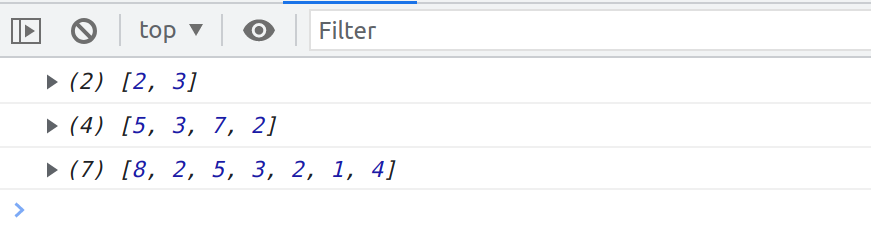As you can see, the function has been called three times giving us three arrays with all the arguments that we passed into the functions. But they are indeed arrays.

Once again, as I said in the beginning the rest syntax takes multiple numbers or multiple values and then packs them all into one array. It does the opposite of the spread operator. With spread operator, we expand, while with the rest syntax, we compress.

Let's now write the logic to actually add all the numbers together.

script.js
``````const add = function (...numbers) {
let sum = 0;

for (let i = 0; i < numbers.length; i++) {
sum += numbers[i];
}

console.log(sum);
};``````We get the expected result. This actually shows that our add function is a working function that can accept any number of parameters, and hopefully, you are not confused by this.

Let's take it to the next level. Consider the below array:

script.js
``const x = [23, 5, 7];``

If we wanted to take these values from the `x` array and call the `add` function with those values, how would we do that? We will use the spread operator, and that's it, just like we learned in the previous lecture.

script.js
``````//Her we PACK them together into an array
const add = function (...numbers) {
// LOGIC
};

const x = [23, 5, 7];

// Here we UNPACK the values (add(23, 5, 7))

The three dots will spread all the elements from the x array individually into the add function. It would be the same as writing manually 23, 5 and 7. This is actually a good example of showing you how the spread operator is the opposite of the rest operator. Because, after the numbers are spread, they will enter the `add` function and then they will immediately be collected into the `numbers` array by the rest parameters.

You could wonder why we are not simply writing an add function that takes an array as an argument, and then we won't need all of this. Well, it's way better to do it using rest parameters because then the function can accept both an array by doing `add(...x)` and all the single values by doing `add(1,2,3,4)`.

Also, it feels more natural to pass as many arguments as we want to add together into the function without dealing with arrays if we don't want to.

To finish this lecture, let's use rest parameters in our restaurant example to see some edge cases. Let's add another method to our `restaurant` object to order Pizza.

script.js
``````const restaurant = {
// OTHER PROPERTIES AND METHODS

orderPizza: function () {

},
};``````

Let say Pizzas need to have at least one ingredient, but the other ingredients are optional, which is a perfect scenario for rest parameters.

script.js
``````const restaurant = {
// OTHER PROPERTIES AND METHODS

orderPizza: function (mainIngredient, ...otherIngredients) {
console.log(mainIngredient);
console.log(otherIngredients);
},
};

restaurant.orderPizza('mushrooms', 'onions', 'olives', 'spinach');``````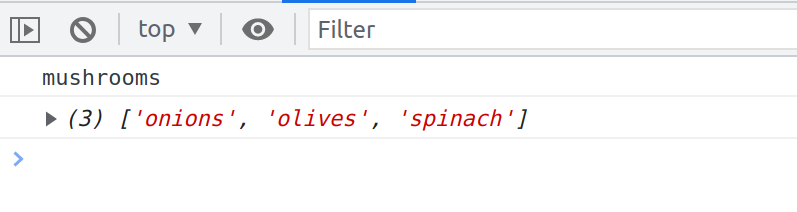You can see that we get mushrooms, which is this first, or main ingredient and then we get an array of all the remaining ingredients that we passed in which is the result of the rest arguments.

Again, the first argument `pizza` was stored in the `mainIngredient` parameter in our `orderPizza` method. Then all the remaining arguments that were passed in were simply stored in the array `otherIngredients` by the rest parameter syntax.

And of course we counld simply just define one argument

script.js
``restaurant.orderPizza('mushrooms');``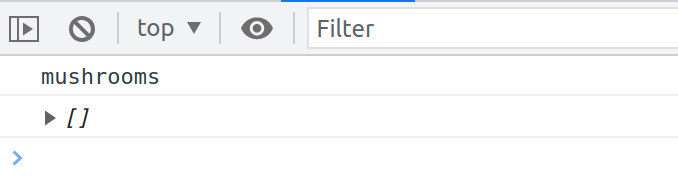As you can see, the remaining arguments will simply be put in an empty array because of course there are none and so there is nothing to collect into the array but we still get an empty array that we can work with if we want.

To recap, the spread and rests syntax both look the same, but they work in opposite ways depending on where they are used. The spread operator is used where we would otherwise write values, separated by a comma. On the other hand, the rest pattern is basically used where we would otherwise write variable names separated by commas.

Again, the rest pattern can be used where we would write VARIABLE names, separated by commas and NOT VALUES separated by commas. So it's a subtle distinction, but this is how you know when and where to use spread and rest.